• 常微分方程数值求解;主要内容 数值求解常微分方程组函数概述 非刚性/刚性常微分方程问题求解 隐式微分方程组求解 微分代数方程(DAE)与延迟微分方程(DDE)求解 边值问题求解 ;第一节数值求解常微分方程组函数概述;一...
• 常微分方程数值求解常微分方程数值求解的一般概念常微分方程数值求解的一般概念常微分方程数值求解函数常微分方程数值求解函数求常微分方程数值解的函数举几个个栗子例一例二刚性问题举个例子 常微分方程数值求解的...


常微分方程数值求解
常微分方程数值求解的一般概念常微分方程数值求解的一般概念

常微分方程数值求解函数常微分方程数值求解函数求常微分方程数值解的函数举几个个栗子例一例二

刚性问题举个例子

常微分方程数值求解的一般概念
常微分方程数值求解的一般概念
求解微分方程概念 求解常微分方程初值问题就是寻找函数y(t)使之满足如下方程： y’=f(t,y)，t0≤t≤b ,y(t0)=y0 所谓其数值解法，就是求y(t)在离散结点tn处的函数近似值yn的方法，yn≈y(xn)。 这些近似值称为常微分方程初值问题的数值解。相邻两个结点之间的距离称为步长。  两种方法：
常微分方程数值求解函数
常微分方程数值求解函数
调用格式 [t,y]=solver(filename,tspan,y0,option)
t和y分别给出时间向量和相应的数值解。solver为求常微分方程数值解 的函数（即下面表格中的函数）。filename是定义f(t，y)的函数名，该函数必须返回一个列向量。tspan形式为[t0，tf]，表示求解区间。y0是初始状态向量。Option是可选参数， 用于设置求解属性，常用的属性包括相对误差值RelTol(默认值是10-3)和绝对误 差值AbsTol(默认值是10-6)。
常微分方程数值求解函数的统一命名格式： odennxx
ode是Ordinary Differential Equation的缩写，是常微分方程的意思。nn是数字，代表所用方法的阶数。 例如，ode23采用2阶龙格-库塔（Runge-Kutta）算法，用3阶公式做误差估计来调节步长，具有低等精度。 ode45采用4阶龙格-库塔算法，用5阶公式做误差估计来调节步长，具有中 等精度。xx是字母，用于标注方法的专门特征。例如，ode15s、ode23s中的“s” 代表（Stiff），表示函数适用于刚性方程。
求常微分方程数值解的函数举几个个栗子
例一f=@(t,y) (y^2-t-2)/4/(t+1);
[t,y]=ode23(f,[0,10],2);
y1=sqrt(t+1)+1;%精确计算
plot(t,y,'b:',t,y1,'r');%绘图比较一下精确计算与数值求解的结果，从图形可以看出拟合较好例二f=@(t,x) [-2,0;0,1]*[x(2);x(1)];
[t,x]=ode45(f,[0,20],[1,0]); %四个未知数
subplot(2,2,1);plot(t,x(:,2));
subplot(2,2,2);plot(x(:,2),x(:,1));刚性问题
有一类常微分方程，其解的分量有的变化很快，有的变化很慢，且相差悬殊，这就是所谓的刚性问题(Stiff)。 对于刚性问题，数值解算法必须取很小步长才能获得满意的结果，导致计算 量会大大增加。解决刚性问题需要有专门方法。非刚性算法可以求解刚性问 题，只不过需要很长的计算时间。
举个例子
假如点燃一个火柴，火焰球迅速增大直至某个临界体积，然后，维持这一体积不变，原因是火焰球内部燃烧耗费的氧气和从球表面所获氧气达到平衡。其简化模型如下：其中，y(t)代表火焰球半径，初始半径是λ，它很小。分析λ的大小对方程求解过程的影响。 这里列出两个方法，通过对比时间长短体会刚性问题的特殊解法。
lamada较大采用非刚性解法
f=@(t,y) y^2-y^3;
tic;%记录开始时间
[t,y]=ode45(f,[0,2/lamda],lamda);%非刚性解法
toc ;%记录结束时间
disp(['ode45计算的点数' num2str(length(t))]);
结果：
时间已过 0.061455 秒。%时间较短，说明此时没有较明显的刚性
ode45计算的点数157

lamada较小，两种方法对比
非刚性解法：
lamda=1e-5; %lamda特别小
f=@(t,y) y^2-y^3;
tic;
[t,y]=ode45(f,[0,2/lamda],lamda);
toc;
disp(['ode45计算的点数' num2str(length(t))]);
结果：
时间已过 0.230707 秒。
ode45计算的点数120565%时间长，点数多表明明显的钢性特征

刚性解法：
lamda=1e-5;
f=@(t,y) y^2-y^3;
tic;
[t,y]=ode15s(f,[0,2/lamda],lamda);
toc
disp(['ode15s计算的点数' num2str(length(t))])
结果：
时间已过 0.044472 秒。
ode15s计算的点数98

展开全文matlab 程序人生
• 本节将介绍常微分方程初值问题的数值求解，主要内容分为三个部分：常微分方程数值求解的概念、求解函数及刚性问题。 一、常微分方程数值求解的一般概念 首先，凡含有参数，未知函数和未知函数导数 (或微分) 的方程，...
本节将介绍常微分方程初值问题的数值求解，主要内容分为三个部分：常微分方程数值求解的概念、求解函数及刚性问题。 一、常微分方程数值求解的一般概念 首先，凡含有参数，未知函数和未知函数导数 (或微分) 的方程，称为微分方程，有时简称为方程，未知函数是一元函数的微分方程称作常微分方程，未知函数是多元函数的微分方程称作偏微分方程。微分方程中出现的未知函数最高阶导数的阶数，称为微分方程的阶。 常微分方程数值求解，指研究求解初值问题各类数值方法的构造、理论分析与数值实现问题。研究的主要对象为一阶方程组初值问题：其中，其中y=y(x)是未知函数,y(x0)=y0是初值条件,而f(x,y)是给定的二元函数。 所谓其数值解法，就是求y(x)在离散结点xn处的函数近似值yn 的方法 ，yn≈y(xn)。这些近似值称为常微分方程初值问题的数值解。相邻两个结点之间的距离称为步长。 这里主要介绍两种：单步法和多步法 单步法：在计算y(n+1)时只用到前一步的y(n)，因此在有了初值之后就可以逐步往下计算，其代表是龙格- - 库塔（ Runge- - Kutta ） 法 多步法：在计算y(n+1)时，除了用到前一步的值y(n)之外, , 还要用到y(n-p)（ p=1，2 ， … ，k，k＞0）的值, , 即前面的k步。其代表就是亚当斯 (Adams) 法 更多介绍可参见这个链接： https://wenku.baidu.com/view/cafe161b9a6648d7c1c708a1284ac850ad0204fc.html 二、常微分方程求解函数 MATLAB 提供了多个求常微分方程初值问题数值解的函数，一般调用格式为： [t,y]=solver(filename,tspan,y0,option) 其中，t和y分别给出时间向量和相应的数值解。solver为求常微分方程数值解的函数。filename 是定义 f(t ，y) 的函数名，该函数必须返回一个列向量。 tspan 形式为 [t0，tf]，表示求解区间。 y0 是初始状态向量。Option 是可选参数，用于设置求解属性，常用的属性包括相对误差值 RelTol(默认值是10的-3次方)和绝对误差值 AbsTol( ( 默认值是10的-6次方)。 常微分方程数值求解函数的统一命名格式： odennx ode是Ordinary Differential Equation 的缩写，是常微分方程的意思。 nn 是数字，代表所用方法的阶数。例如，ode23采用2阶龙格- - 库塔（ Runge- - Kutta ）算法 ，用3阶公式做误差估计来调节步长，具有低等精度。ode45 采用4阶龙格- - 库塔算 法 ，用5阶公式做误差估计来调节步长，具有中等精度。 x是字母，用于标注方法的专门特征。例如， ode15s 、ode23s 中的“s”代表（ Stiff ），表示函数适用于刚性方程。 下表列出了求常微分方程数值解的函数：
求解函数采用方法适用场合ode232 阶或3阶 Runge- - Kutta 算法，低精度非刚性ode454 阶或5阶 Runge- - Kutta 算法，中精度非刚性ode113Adams 算法，精度可到10的-3次方至10的-6次方非刚性，计算时间比 ode45ode23t梯形算法适度刚性ode15sGear’s 反向数值微分算法，中精度刚性ode23s2阶 Rosebrock 算法，低精度刚性，当精度较低时，计算时间比 ode15sode23tb梯形算法，低精度刚性，当精度较低时，计算时间比 ode15s
先看一个简单例子，y‘=y+3x/x^2，初值y(0)=-2，求解区间为[1,4]。
>> odefun=@(x,y) (y+3*x)/x^2;
tspan=[1 4];
y0=-2;
[x y]=ode45(odefun,tspan,y0)
plot(x,y)二、刚性问题 有一类常微分方程，其解的分量有的变化很快，有的变化很慢，且相差悬殊，这就是所谓的刚性问题 (Stiff) 。 对于刚性问题，数值解算法必须取很小步长才能获得满意的结果，导致计算量会大大增加。解决刚性问题需要有专门方法。非刚性算法可以求解刚性问题，只不过需要很长的计算时间。 例、假如点燃一个火柴，火焰球迅速增大直至某个临界体积，然后，维持这一体积不变，原因是火焰球内部燃烧耗费的氧气和从球表面所获氧气达到平衡。其简化模型如下： y’=y2-y3，y(0)=L，0<=t<=2/L 其中， y(t) 代表火焰球半径，初始半径是λ ，它很小。分析 λ 的大小对方程求解过程的影响。
>>  L=0.01;
f=@(t,y) y^2-y^3;
tic;[ t,y ]=ode45(f,[0,2/L],L); toc
disp (['ode45 计算的点数' num2str(length(t))]);
时间已过 0.003704 秒。
ode45 计算的点数157
>>  L=1e-5;
f=@(t,y) y^2-y^3;
tic;[ t,y ]=ode45(f,[0,2/L],L); toc
disp (['ode45 计算的点数' num2str(length(t))]);
时间已过 1.010016 秒。
ode45 计算的点数120565
>> L=1e-5;
f=@(t,y) y^2-y^3;
tic;[ t,y ]=ode15s(f,[0,2/L],L); toc
disp (['ode45 计算的点数' num2str(length(t))]);
时间已过 0.189977 秒。
ode45 计算的点数98

注：tic 和 toc 函数 用来记录 微分方程求解 命令执行的时间 ，使用 tic 函数启动计时器，使用 toc 函数显示从计时器启动到当前所经历的时间。 上述采用了三种不同方法，可以发现，第一种的运行结果表明这时常微分方程不算很刚性；第二种这时计算时间明显加长，计算的点数剧增，表明这时常微分方程表现为刚性；因此对于刚性问题，我们需要改变求解算法，我们选择以“s”结尾的函数，例如第三种方法他们专门用于求解刚性方程。计算时间大大缩短，计算的点数大大减少。 常微分方程数值解法的研究已发展得相当成熟，理论上也颇为完善，小编由于能力有限，只能了解个大概，本文讲解也就写的是比较基础的一些方面，如果大家有更多需求，可以自己查阅相关资料。
关于MATLAB的学习：
大家可以关注我们的知乎专栏——数据可视化和数据分析中matlab的使用： https://zhuanlan.zhihu.com/c_1131568134137692160
欢迎大家加入我们的MATLAB学习交流群： 953314432 扫码关注我们 发现更多精彩展开全文线性代数
• 数学实验第二次作业_常微分方程数值求解.docx文档
• 常微分方程数值求解1. 常微分方程数值求解的一般概念2. 常微分方程数值求解函数3. 常微分方程数值求解函数统一命名格式4. 刚性问题 1. 常微分方程数值求解的一般概念 2. 常微分方程数值求解函数 [t,y]=solver...


常微分方程数值求解
1. 常微分方程数值求解的一般概念2. 常微分方程数值求解函数3. 常微分方程数值求解函数统一命名格式4. 刚性问题

1. 常微分方程数值求解的一般概念
2. 常微分方程数值求解函数
[t,y]=solver(filename,tspan,y0,option) t：时间向量； y：数值解； filename：定义f(t,y)的函数名，该函数必须返回一个列向量； tspan形式为[t0,tf]，表示求解区间； y0：初始状态向量； option：可选参数，用于设置求解属性，常用的属性包括相对误差值RelTol（默认值为10-3和绝对误差值AbsTol（默认值为10-6）；
3. 常微分方程数值求解函数统一命名格式
odennxx
ode：Ordinary Differential Equation，常微分方程nn：数字，代表所用方法的阶数。 ode23采用2阶龙格-库塔（Runge-Kutta）算法，用3阶公式做误差估计来调节步长，具有低等精度。 ode45采用4阶龙格-库塔算法，用5阶公式做误差估计来调节步长，具有中等精度。xx：字母，用于标注方法的专门特征。 ode15s、ode23s中的“s”代表（Stiff），表示函数适用于刚性方程。e.g.求解微分方程初值问题，并与精确解y(t)=sqrt(t+1)+1，进行比较。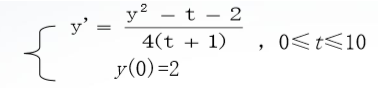>> f=@(t,y)(y^2-t-2)/4/(t+1);
>> [t,y]=ode23(f,[0,10],2);
>> y1=sqrt(t+1)+1;
>> plot(t,y,'b:',t,y1,'r')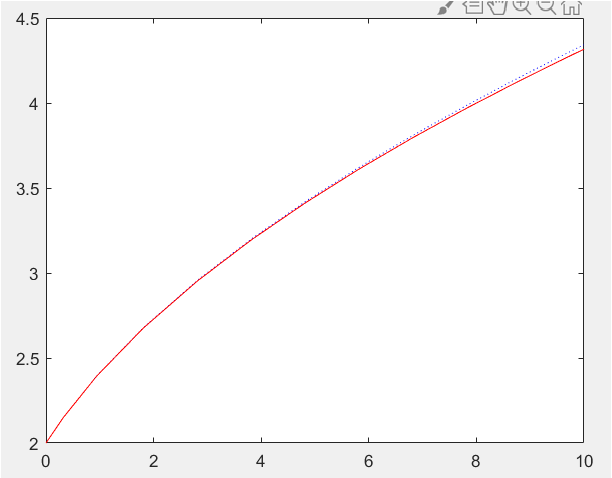e.g.已知一个二阶线性系统的微分方程为：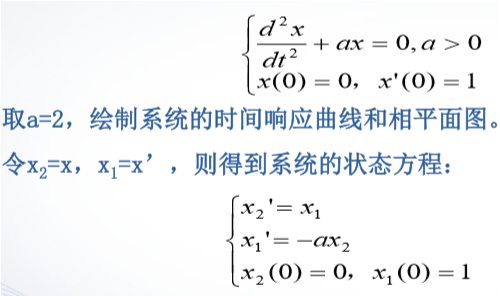>> f=@(t,x) [-2,0;0,1]*[x(2);x(1)];
>> [t,x]=ode45(f,[0,20],[1,0]);
>> subplot(2,2,1);
>> plot(t,x(:,2));
>> subplot(2,2,2);
>> plot(x(:,2),x(:,1));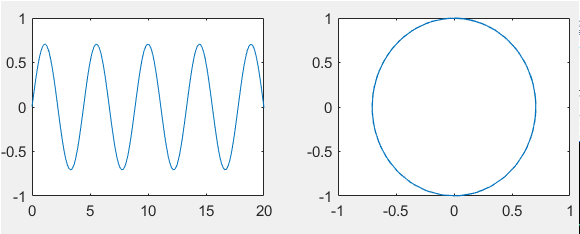4. 刚性问题
有一类常微分方程，其解的分量有的变化很快，有的变化很慢，且相差悬殊，这就是所谓的刚性问题(Stiff)。对于刚性问题，数值解算法必须取很小步长才能获得满意的结果，导致计算量会大大增加。解决刚性问题需要有专门方法。非刚性算法可以求解刚性问题，只不过需要很长的计算时间。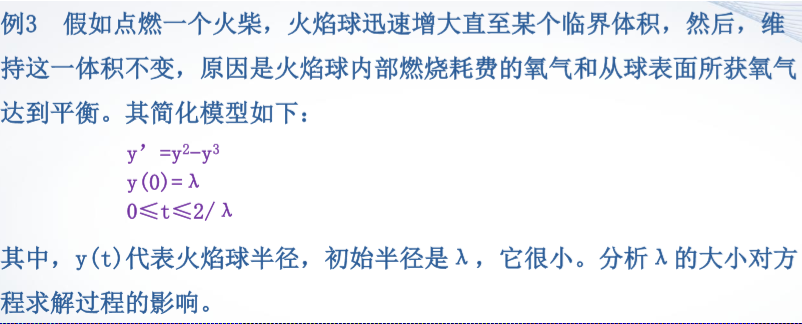>> lambda=0.01;
>> f=@(t,y) y^2-y^3;
>> tic;
>> [t,y]=ode45(f,[0,2/lambda],lambda);
>> toc
时间已过 32.701709 秒。
>> disp(['ode45计算的点数' num2str(length(t))]);
ode45计算的点数157
%tic和toc函数用来记录微分方程求解命令执行的时间，使用tic函数启动计时器，使用toc函数显示从计时器启动到当前所经历的时间。最后还输出计算的点数，运行结果表明这时常微分方程不算很刚性。

>> lambda=1e-5;
>> f=@(t,y) y^2-y^3;
>> tic;
>> f=@(t,y) y^2-y^3;
>> toc
时间已过 7.096559 秒。
>> disp(['ode45计算的点数' num2str(length(t))]);
ode45计算的点数157
这时计算时间明显加长，计算的点数剧增，表明这时常微分方程表现为刚性

>> lambda=1e-5;
>> f=@(t,y) y^2-y^3;
>> tic;
>> [t,y]=ode15s(f,[0,2/lambda],lambda);
>> toc
时间已过 8.699999 秒。
>> disp(['ode15s计算的点数' num2str(length(t))]);
ode15s计算的点数98
对于刚性问题，选择以“s”结尾的函数，专门用于求解刚性方程。计算时间大大缩短，计算的点数大大减少。
相同程序，每次的计算时间都不相同

展开全文• 常微分方程数值求解的一般概念 常微分方程数值求解函数 刚性问题 常微分方程数值求解的一般概念 求解常微分方程初值问题就是寻找函数y(t)使之满足如下方程： 所谓其数值解法，就是求y(t)在离散结点t0处的函数近似...
本次讨论取材于中南大学《科学计算与matlab语言》内容为常微分方程数值解法
常微分方程数值求解的一般概念常微分方程数值求解函数刚性问题
常微分方程数值求解的一般概念
求解常微分方程初值问题就是寻找函数y(t)使之满足如下方程：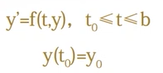所谓其数值解法，就是求y(t)在离散结点t0处的函数近似值y0的方法
常微分方程的求解函数
函数调用格式为：
[t,y]=solver(filename,tspan,y0,option)

其中，t和y分别给出时间向量和相应的数值解；solver为求常微分方程数值解的函数；filename是定义f(t,y)的函数名，该函数必须返回一个列向量；tspan形式为[t0,tf]，表示求解区间；y0是初始状态向量;Option是可选参数,用于设置求解属性.
常微分方程数值求解函数的统一命名格式：
odennxx

其中ode是Ordinary Differential Equation的缩写，是常微分方程的意思；nn是数字，代表所用方法的阶数；xx是字母，用于标注方法的专门特征。>> f=@(t,y) (y^2-t-2)/4/(t+1);
>> [t,y]=ode23(f,[0,10],2);
>> y1=sqrt(t+1)+1;
>> plot(t,y,'b:',t,y1,'r');
>>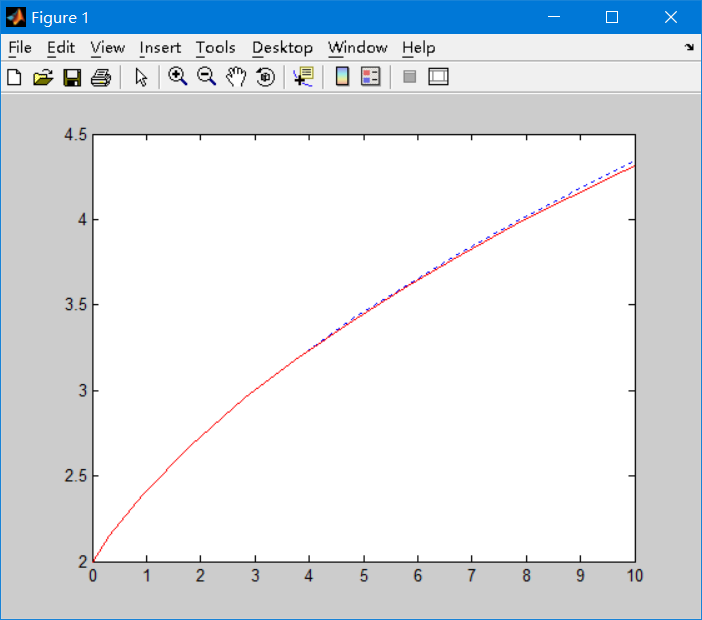例2 已知一个二阶线性系统的微分方程为：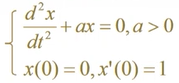取a=2，绘制系统的时间响应曲线和相平面图。 令x2=x,x1=x’，则得到系统的状态方程：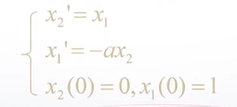f=@(t,x) [-2,0;0,1]*[x(2);x(1)];
[t,x]=ode45(f,[0,20],[1,0]);
subplot(2,2,1);plot(t,x(:,2));
subplot(2,2,2);plot(x(:,2),x(:,1));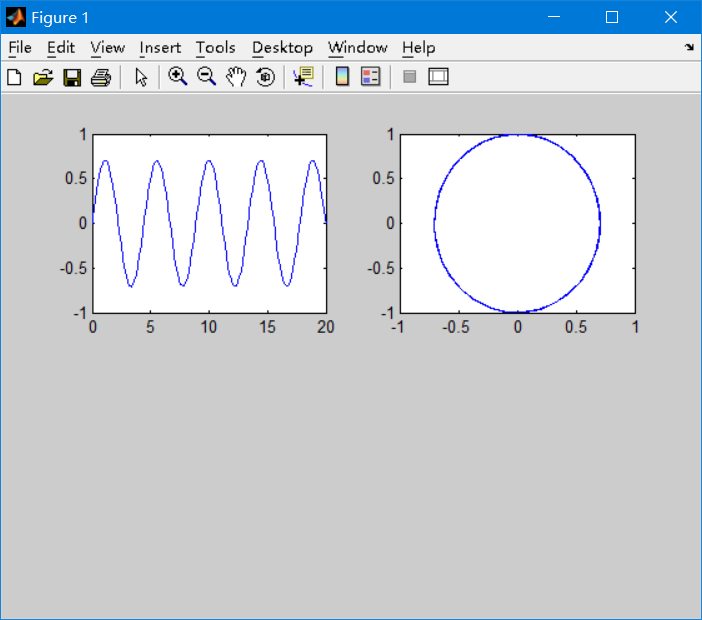刚性问题
有一类常微分方程，其解的分量有的变化很快，有的变化很慢，且相差悬殊，这就是所谓的刚性问题(Stiff) 例3 假如点燃一个火柴，火焰球迅速增大直至某个临界体积，然后，维持这一体积不变，其原因是火焰球内部燃烧耗费的氧气和从球表面所或氧气达到平衡。主要观看λ值得大小，是否会真正影响问题模型！
>> lamda=0.01;
>> f=@(t,y) y^2-y^3;
>> tic;[t,y]=ode45(f,[0,2/lamda],lamda);toc
Elapsed time is 0.034000 seconds.
>> disp(['ode45计算的点数' num2str(length(t))]);
ode45计算的点数157
>>

时间已过0.034000秒 ode45计算的点数157
>> lamda=1e-5;
>> f=@(t,y) y^2-y^3;
>> tic;[t,y]=ode45(f,[0,2/lamda],lamda);toc
Elapsed time is 5.690000 seconds.
>> disp(['ode45计算的点数' num2str(length(t))]);
ode45计算的点数120565
>>

不同的λ值，式子所用的秒数不同，问题瞬间变成刚性，直接换用ode15s，再去计算！直接秒出答案！
>> tic;[t,y]=ode15s(f,[0,2/lamda],lamda);toc
Elapsed time is 0.348000 seconds.
>> disp(['ode15s计算的点数' num2str(length(t))]);
ode15s计算的点数98
>>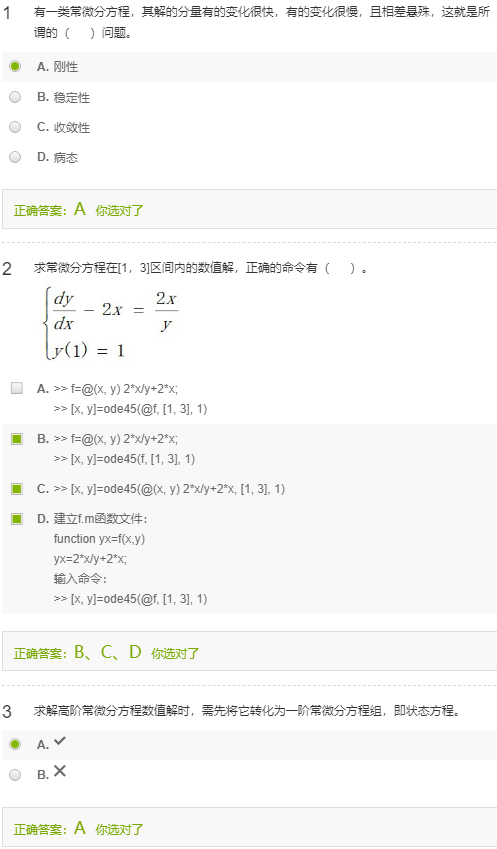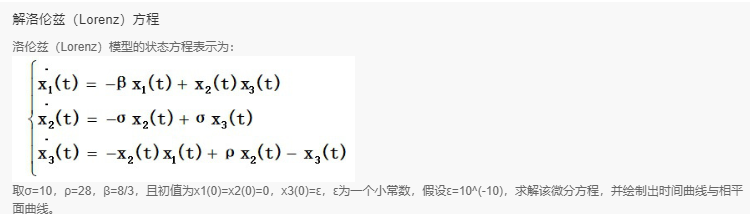>> f=@(t,x) [-8/3*x(1)+x(2)*x(3);-10*x(2)+10*x(3);-x(2)*x(1)+28*x(2)-x(3)];

>> [t,x]=ode45(f,[0,50],[0,0,10^(-10)]);

>> plot(t,x)


展开全文• 这里只考虑最为简单的一种常微分方程 dydx=f(x,y)\frac{dy}{dx} = f(x,y)dxdy​=f(x,y) 然后这里的实例都是以下面这个方程来做展示的。 dydx=y∗(1−y2)\frac{dy}{dx} = y*(1-y^2)dxdy​=y∗(1−y2) 初值给定 y...Python
• 本文为印度ROURKELA国立技术研究所（作者：Pramod Kumar Parida）的硕士论文，共29页。本文介绍了利用人工神经网络求解常微分方程的方法。在不直接使......